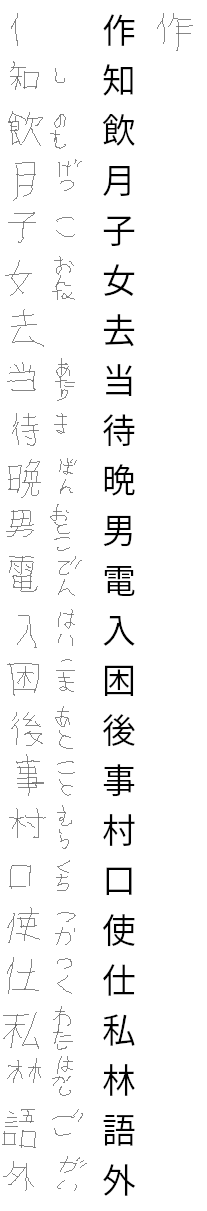## Learning to Read and Write Kanji Row 8

I've been learning Japanese again. This is looking like it's going to be a series where I show my progress (see the bottom of the post for previous episodes). To show my progress, see the test below and the list of kanji is available below as well.As with the previous row, most of these are from row 8 and some of them are from earlier rows. I made 3 mistakes which is higher than usual, but because I was able to draw all the kanji from row 8 correctly, I am moving on. I forgot the reading for 去 (さ) and misremembered the reading for 使 (つか). Both those I'll have plenty of time to learn. The only kanji I failed to draw is one I had trouble with before (作). Because of repeated problems with this kanji, it will get extra attention. If we do the math, what is the likelihood of getting 去 and 使 at some point in the future? This is actually pretty difficult math; something I am sure to get incorrect. But we can approximate an answer by seeing how many options there are and how many are going to be picked from those options. There are 112 kanji in row 1-8. We pick 24 kanji for the test, 14 will come from row 9 for the row 9 test leaving 10 from rows 1-8. So picking randomly we have a (1/112 + 1/111 + 1/110 + ... + 1/103) chance.

``````
sum([1/x for x in list(range(112, 102, -1))])
0.0930897493743004
``````

So that's the probability of getting one kanji, but our problem is about 2 kanji, right? So we multiply by 2 to get our approximate probability. So there's around a 19% chance that I'll get one in row 9 test. If we were to finish the math up to row 21, it's high likelihood of getting both those kanji. What are the odds of getting both the kanji in row 9 test? For this we have to multiply the odds of getting one with the odds of getting the other. We have those values, so 0.093*0.093 the odds are 0.00867, not nearly as good. But over 12 more tests, the odds will grow some.

I'll hopefully post all tests as I pass them. So far it's been quite efficient and has improved my outlook on this project as an actual long-term valuable skill I can use.

Javantea out.